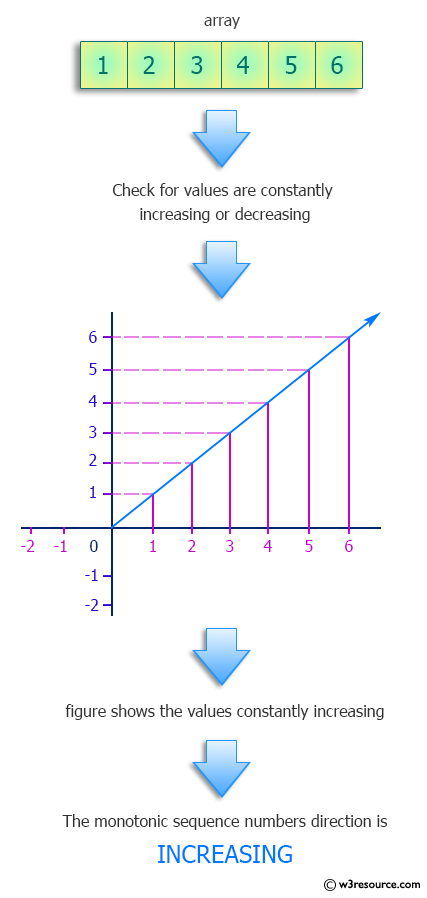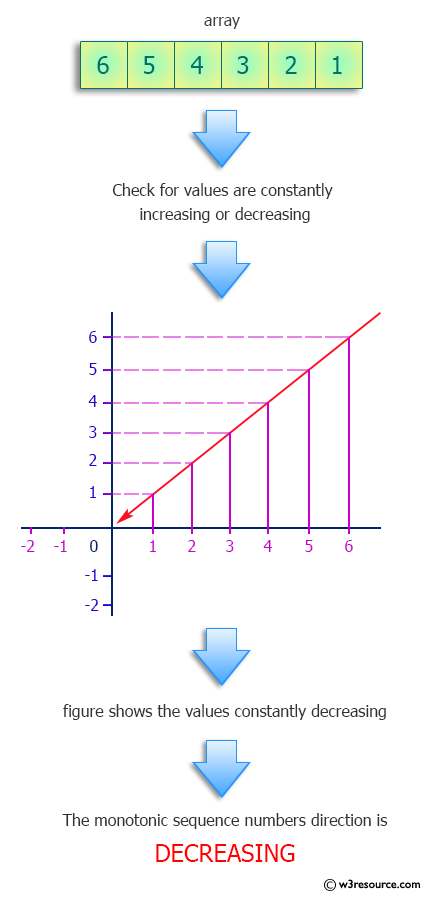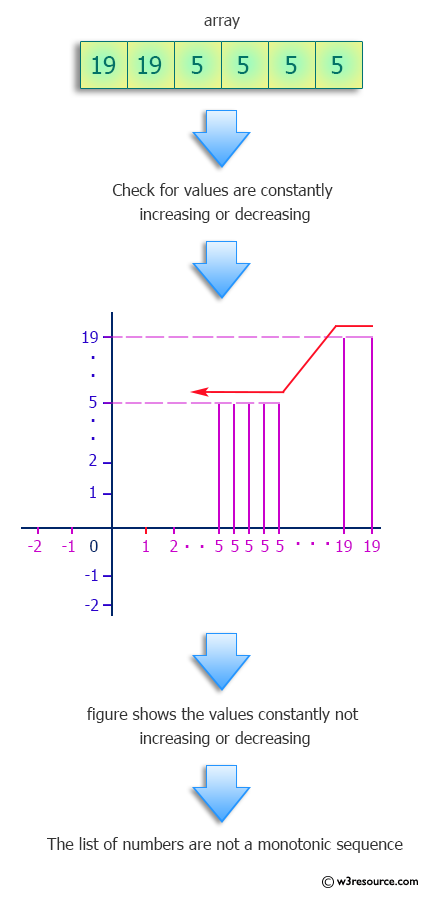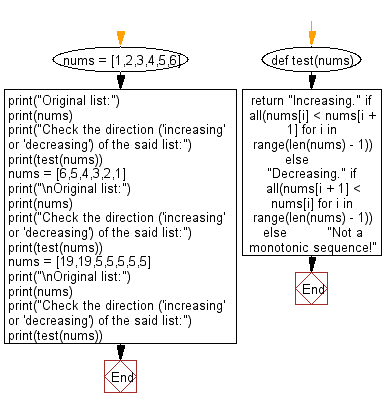﻿ Python: Determine the direction ('increasing' or 'decreasing') of monotonic sequence numbers - w3resource# Python: Determine the direction ('increasing' or 'decreasing') of monotonic sequence numbers

## Python Programming Puzzles: Exercise-20 with Solution

Monotonic sequences are sequences, which constantly increase or constantly decrease.
Write a Python program to determine the direction ('increasing' or 'decreasing') of monotonic sequence numbers.

```Input:
[1, 2, 3, 4, 5, 6]
Output:
Increasing.

Input:
[6, 5, 4, 3, 2, 1]
Output:
Decreasing.

Input:
[19, 19, 5, 5, 5, 5, 5]
Output:
Not a monotonic sequence!
```

Pictorial Presentation:Sample Solution:

Python Code:

``````#License: https://bit.ly/3oLErEI
def test(nums):
return "Increasing." if all(nums[i] < nums[i + 1] for i in range(len(nums) - 1)) else \
"Decreasing." if all(nums[i + 1] < nums[i] for i in range(len(nums) - 1)) else \
"Not a monotonic sequence!"
nums = [1,2,3,4,5,6]
print("Original list:")
print(nums)
print("Check the direction ('increasing' or 'decreasing') of the said list:")
print(test(nums))
nums = [6,5,4,3,2,1]
print("\nOriginal list:")
print(nums)
print("Check the direction ('increasing' or 'decreasing') of the said list:")
print(test(nums))
nums = [19,19,5,5,5,5,5]
print("\nOriginal list:")
print(nums)
print("Check the direction ('increasing' or 'decreasing') of the said list:")
print(test(nums))
``````

Sample Output:

```Original list:
[1, 2, 3, 4, 5, 6]
Check the direction ('increasing' or 'decreasing') of the said list:
Increasing.

Original list:
[6, 5, 4, 3, 2, 1]
Check the direction ('increasing' or 'decreasing') of the said list:
Decreasing.

Original list:
[19, 19, 5, 5, 5, 5, 5]
Check the direction ('increasing' or 'decreasing') of the said list:
Not a monotonic sequence!
```

Flowchart:## Visualize Python code execution:

The following tool visualize what the computer is doing step-by-step as it executes the said program:

Python Code Editor :

Have another way to solve this solution? Contribute your code (and comments) through Disqus.

What is the difficulty level of this exercise?

Test your Programming skills with w3resource's quiz.

﻿

## Python: Tips of the Day

Clamps num within the inclusive range specified by the boundary values x and y:

Example:

```def tips_clamp_num(num,x,y):
return max(min(num, max(x, y)), min(x, y))
print(tips_clamp_num(2, 4, 6))
print(tips_clamp_num(1, -1, -6))
```

Output:

```4
-1
```